Anúncio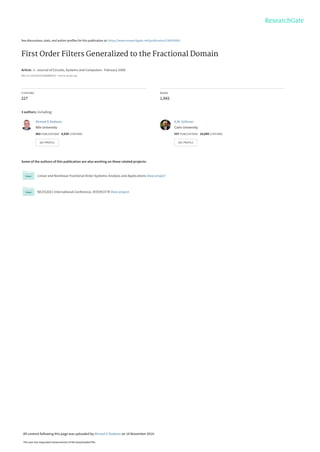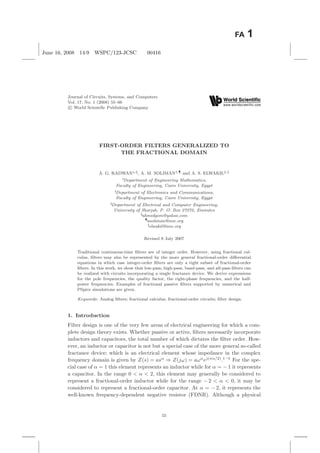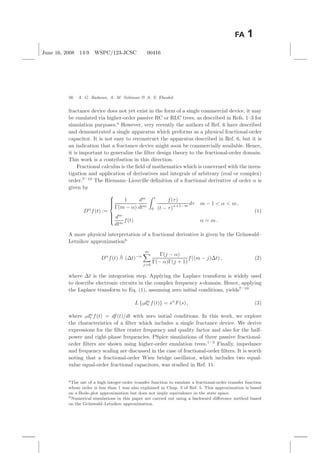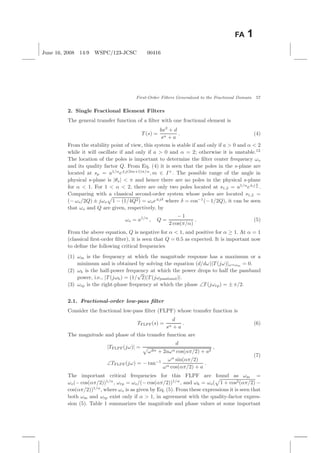Anúncio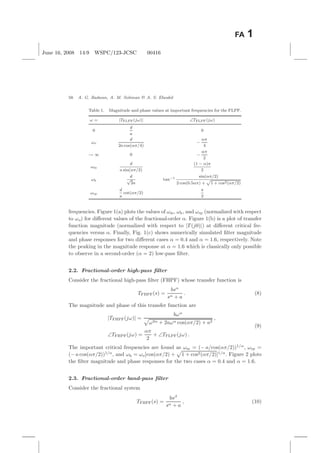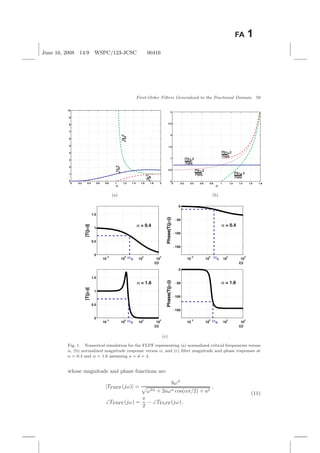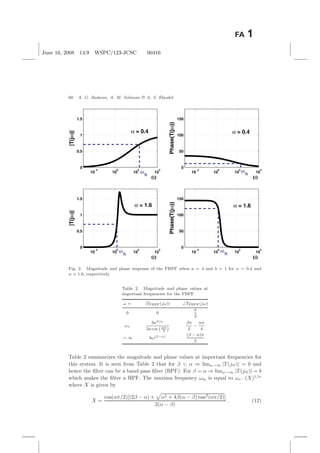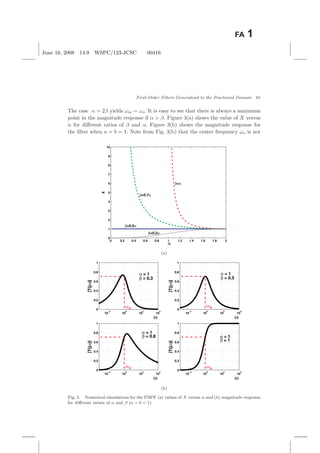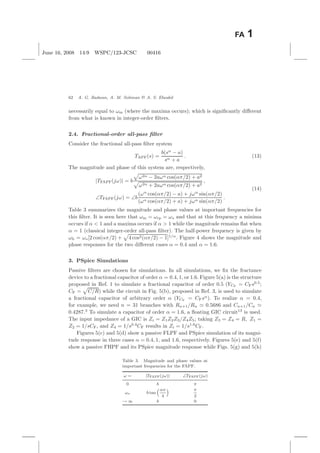Anúncio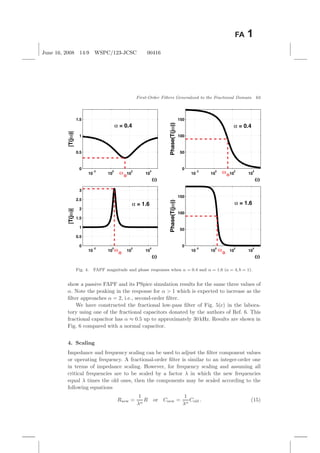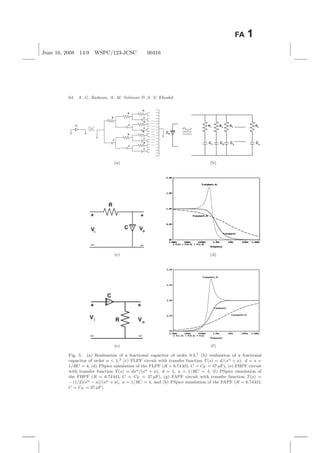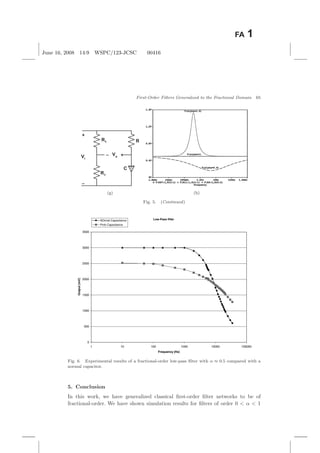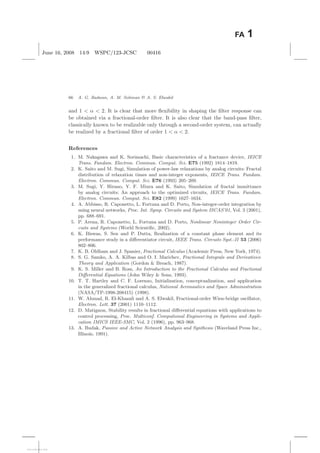Próximos SlideShares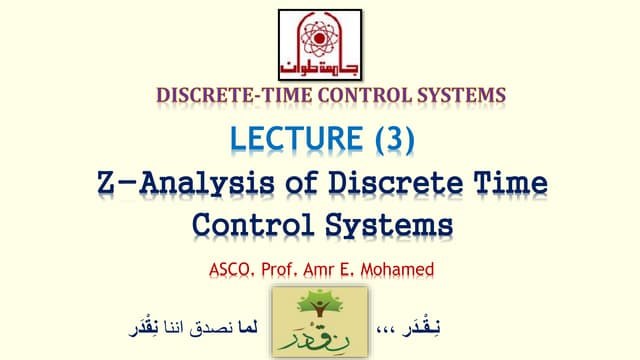Dcs lec03 - z-analysis of discrete time control systems
Carregando em ... 3
1 de 13
Anúncio

### 2008-FIRST-ORDERFILTERSGENERALIZEDJCSC.pdf

1. See discussions, stats, and author profiles for this publication at: https://www.researchgate.net/publication/230635692 First Order Filters Generalized to the Fractional Domain Article  in  Journal of Circuits, Systems and Computers · February 2008 DOI: 10.1142/S0218126608004162 · Source: dx.doi.org CITATIONS 227 READS 1,942 3 authors, including: Some of the authors of this publication are also working on these related projects: Linear and Nonlinear Fractional Order Systems: Analysis and Applications View project NILES2021 International Conference, IEEE#53778 View project Ahmed G Radwan Nile University 463 PUBLICATIONS   8,939 CITATIONS    SEE PROFILE A.M. Soliman Cairo University 557 PUBLICATIONS   10,689 CITATIONS    SEE PROFILE All content following this page was uploaded by Ahmed G Radwan on 14 November 2014. The user has requested enhancement of the downloaded file.
2. June 16, 2008 14:9 WSPC/123-JCSC 00416 Journal of Circuits, Systems, and Computers Vol. 17, No. 1 (2008) 55–66 c World Scientiﬁc Publishing Company FIRST-ORDER FILTERS GENERALIZED TO THE FRACTIONAL DOMAIN A. G. RADWAN∗,§, A. M. SOLIMAN†,¶ and A. S. ELWAKIL‡, ∗Department of Engineering Mathematics, Faculty of Engineering, Cairo University, Egypt †Department of Electronics and Communications, Faculty of Engineering, Cairo University, Egypt ‡Department of Electrical and Computer Engineering, University of Sharjah, P. O. Box 27272, Emirates §ahmedgom@yahoo.com ¶asoliman@ieee.org elwakil@ieee.org Revised 8 July 2007 Traditional continuous-time ﬁlters are of integer order. However, using fractional cal- culus, ﬁlters may also be represented by the more general fractional-order diﬀerential equations in which case integer-order ﬁlters are only a tight subset of fractional-order ﬁlters. In this work, we show that low-pass, high-pass, band-pass, and all-pass ﬁlters can be realized with circuits incorporating a single fractance device. We derive expressions for the pole frequencies, the quality factor, the right-phase frequencies, and the half- power frequencies. Examples of fractional passive ﬁlters supported by numerical and PSpice simulations are given. Keywords: Analog ﬁlters; fractional calculus; fractional-order circuits; ﬁlter design. 1. Introduction Filter design is one of the very few areas of electrical engineering for which a com- plete design theory exists. Whether passive or active, ﬁlters necessarily incorporate inductors and capacitors, the total number of which dictates the ﬁlter order. How- ever, an inductor or capacitor is not but a special case of the more general so-called fractance device; which is an electrical element whose impedance in the complex frequency domain is given by Z(s) = asα ⇒ Z(jω) = aωα ej(πα/2) .1–5 For the spe- cial case of α = 1 this element represents an inductor while for α = − 1 it represents a capacitor. In the range 0 α 2, this element may generally be considered to represent a fractional-order inductor while for the range − 2 α 0, it may be considered to represent a fractional-order capacitor. At α = − 2, it represents the well-known frequency-dependent negative resistor (FDNR). Although a physical 55
3. June 16, 2008 14:9 WSPC/123-JCSC 00416 56 A. G. Radwan, A. M. Soliman A. S. Elwakil fractance device does not yet exist in the form of a single commercial device, it may be emulated via higher-order passive RC or RLC trees, as described in Refs. 1–3 for simulation purposes.a However, very recently the authors of Ref. 6 have described and demonstrated a single apparatus which preforms as a physical fractional-order capacitor. It is not easy to reconstruct the apparatus described in Ref. 6, but it is an indication that a fractance device might soon be commercially available. Hence, it is important to generalize the ﬁlter design theory to the fractional-order domain. This work is a contribution in this direction. Fractional calculus is the ﬁeld of mathematics which is concerned with the inves- tigation and application of derivatives and integrals of arbitrary (real or complex) order.7–10 The Riemann–Liouville deﬁnition of a fractional derivative of order α is given by Dα f(t) :=          1 Γ(m − α) dm dtm t 0 f(τ) (t − τ) α+1−m dτ m − 1 α m , dm dtm f(t) α = m . (1) A more physical interpretation of a fractional derivative is given by the Grünwald– Letnikov approximationb Dα f(t) (∆t)−α m j=0 Γ(j − α) Γ(− α)Γ(j + 1) f((m − j)∆t) , (2) where ∆t is the integration step. Applying the Laplace transform is widely used to describe electronic circuits in the complex frequency s-domain. Hence, applying the Laplace transform to Eq. (1), assuming zero initial conditions, yields7–10 L {0dα t f(t)} = sα F(s) , (3) where 0dα t f(t) = df(t)/dt with zero initial conditions. In this work, we explore the characteristics of a ﬁlter which includes a single fractance device. We derive expressions for the ﬁlter center frequency and quality factor and also for the half- power and right-phase frequencies. PSpice simulations of three passive fractional- order ﬁlters are shown using higher-order emulation trees.1–3 Finally, impedance and frequency scaling are discussed in the case of fractional-order ﬁlters. It is worth noting that a fractional-order Wien bridge oscillator, which includes two equal- value equal-order fractional capacitors, was studied in Ref. 11. aThe use of a high integer-order transfer function to emulate a fractional-order transfer function whose order is less than 1 was also explained in Chap. 3 of Ref. 5. This approximation is based on a Bode-plot approximation but does not imply equivalence in the state space. bNumerical simulations in this paper are carried out using a backward diﬀerence method based on the Grünwald–Letnikov approximation.
4. June 16, 2008 14:9 WSPC/123-JCSC 00416 First-Order Filters Generalized to the Fractional Domain 57 2. Single Fractional Element Filters The general transfer function of a ﬁlter with one fractional element is T (s) = bsβ + d sα + a . (4) From the stability point of view, this system is stable if and only if a 0 and α 2 while it will oscillate if and only if a 0 and α = 2; otherwise it is unstable.12 The location of the poles is important to determine the ﬁlter center frequency ωo and its quality factor Q. From Eq. (4) it is seen that the poles in the s-plane are located at sp = a1/α e±j(2m+1)π/α , m ∈ I+ . The possible range of the angle in physical s-plane is |θs| π and hence there are no poles in the physical s-plane for α 1. For 1 α 2, there are only two poles located at s1,2 = a1/α e±j π α . Comparing with a classical second-order system whose poles are located s1,2 = (− ωo/2Q) ± jωo 1 − (1/4Q2) = ωoe±jδ where δ = cos−1 (− 1/2Q), it can be seen that ωo and Q are given, respectively, by ωo = a1/α , Q = − 1 2 cos(π/α) . (5) From the above equation, Q is negative for α 1, and positive for α ≥ 1. At α = 1 (classical ﬁrst-order ﬁlter), it is seen that Q = 0.5 as expected. It is important now to deﬁne the following critical frequencies (1) ωm is the frequency at which the magnitude response has a maximum or a minimum and is obtained by solving the equation (d/dω)|T (jω)|ω=ωm = 0. (2) ωh is the half-power frequency at which the power drops to half the passband power, i.e., |T (jωh) = (1/ √ 2)|T (jωpassband)|. (3) ωrp is the right-phase frequency at which the phase ∠T (jωrp) = ± π/2. 2.1. Fractional-order low-pass filter Consider the fractional low-pass ﬁlter (FLPF) whose transfer function is TFLPF(s) = d sα + a . (6) The magnitude and phase of this transfer function are |TFLPF(jω)| = d ω2α + 2aωα cos(απ/2) + a2 , ∠TFLPF(jω) = − tan−1 ωα sin(απ/2) ωα cos(απ/2) + a . (7) The important critical frequencies for this FLPF are found as ωm = ωo(− cos(απ/2))1/α , ωrp = ωo/(− cos(απ/2))1/α , and ωh = ωo( 1 + cos2(απ/2) − cos(απ/2))1/α , where ωo is as given by Eq. (5). From these expressions it is seen that both ωm and ωrp exist only if α 1, in agreement with the quality-factor expres- sion (5). Table 1 summarizes the magnitude and phase values at some important
5. June 16, 2008 14:9 WSPC/123-JCSC 00416 58 A. G. Radwan, A. M. Soliman A. S. Elwakil Table 1. Magnitude and phase values at important frequencies for the FLPF. ω = |TFLPF(jω)| ∠TFLPF(jω) 0 d a 0 ωo d 2a cos(απ/4) − απ 4 → ∞ 0 − απ 2 ωm d a sin(απ/2) (1 − α)π 2 ωh d √ 2a tan−1 sin(απ/2) 2 cos(0.5απ) + p 1 + cos2(απ/2) ωrp d a cot(απ/2) π 2 frequencies. Figure 1(a) plots the values of ωm, ωh, and ωrp (normalized with respect to ωo) for diﬀerent values of the fractional-order α. Figure 1(b) is a plot of transfer function magnitude (normalized with respect to |T (j0)|) at diﬀerent critical fre- quencies versus α. Finally, Fig. 1(c) shows numerically simulated ﬁlter magnitude and phase responses for two diﬀerent cases α = 0.4 and α = 1.6, respectively. Note the peaking in the magnitude response at α = 1.6 which is classically only possible to observe in a second-order (α = 2) low-pass ﬁlter. 2.2. Fractional-order high-pass filter Consider the fractional high-pass ﬁlter (FHPF) whose transfer function is TFHPF(s) = bsα sα + a . (8) The magnitude and phase of this transfer function are |TFHPF(jω)| = bωα ω2α + 2aωα cos(απ/2) + a2 , ∠TFHPF(jω) = απ 2 + ∠TFLPF(jω) . (9) The important critical frequencies are found as ωm = (− a/cos(απ/2))1/α , ωrp = (− a cos(απ/2))1/α , and ωh = ωo[cos(απ/2) + 1 + cos2(απ/2)]1/α . Figure 2 plots the ﬁlter magnitude and phase responses for the two cases α = 0.4 and α = 1.6. 2.3. Fractional-order band-pass filter Consider the fractional system TFBPF(s) = bsβ sα + a , (10)
6. June 16, 2008 14:9 WSPC/123-JCSC 00416 First-Order Filters Generalized to the Fractional Domain 59 0 0.2 0.4 0.6 0.8 1 1.2 1.4 1.6 1.8 2 0 1 2 3 4 5 6 7 8 9 10 ωrp o ω ωh o ω ωm ωo α 0 0.2 0.4 0.6 0.8 1 1.2 1.4 1.6 1.8 0 0.5 1 1.5 2 2.5 3 |T(jω )| o |T(j0)| |T(jω )| h |T(j0)| |T(jω )| m |T(j0)| |T(jω )| rp |T(j0)| α (a) (b) 10 −2 10 0 10 2 10 4 0 0.5 1 1.5 ω |T(jω)| α = 0.4 10 −2 10 0 10 2 10 4 −150 −100 −50 0 ω Phase(T(jω)) α = 0.4 10 −2 10 0 10 2 10 4 0 0.5 1 1.5 ω |T(jω)| α = 1.6 10 −2 10 0 10 2 10 4 −150 −100 −50 0 ω Phase(T(jω)) α = 1.6 ωh ωh ωh ωh (c) Fig. 1. Numerical simulation for the FLPF representing (a) normalized critical frequencies versus α, (b) normalized magnitude response versus α, and (c) ﬁlter magnitude and phase responses at α = 0.4 and α = 1.6 assuming a = d = 4. whose magnitude and phase functions are |TFBPF(jω)| = bωβ ω2α + 2aωα cos(απ/2) + a2 , ∠TFBPF(jω) = π 2 − ∠TFLPF(jω) . (11)
7. June 16, 2008 14:9 WSPC/123-JCSC 00416 60 A. G. Radwan, A. M. Soliman A. S. Elwakil 10 −2 10 0 10 2 10 4 0 0.5 1 1.5 ω |T(jω)| α = 0.4 10 −2 10 0 10 2 10 4 0 50 100 150 ω Phase(T(jω)) α = 0.4 10 −2 10 0 10 2 10 4 0 0.5 1 1.5 ω |T(jω)| α = 1.6 10 −2 10 0 10 2 10 4 0 50 100 150 ω Phase(T(jω)) α = 1.6 ωh ωh ωh ωh Fig. 2. Magnitude and phase response of the FHPF when a = 4 and b = 1 for α = 0.4 and α = 1.6, respectively. Table 2. Magnitude and phase values at important frequencies for the FBPF. ω = |TFBPF(jω)| ∠TFBPF(jω) 0 0 π 2 ωo baβ/α 2a cos `απ 4 ´ βπ 2 − απ 4 → ∞ bω(β−α) (β − α)π 2 Table 2 summarizes the magnitude and phase values at important frequencies for this system. It is seen from Table 2 that for β α ⇒ limω→∞ |T (jω)| = 0 and hence the ﬁlter can be a band-pass ﬁlter (BPF). For β = α ⇒ limω→∞ |T (jω)| = b which makes the ﬁlter a HPF. The maxima frequency ωm is equal to ωo · (X)1/α where X is given by X = cos(απ/2)[(2β − α) + α2 + 4β(α − β) tan2 (απ/2)] 2(α − β) . (12)
8. June 16, 2008 14:9 WSPC/123-JCSC 00416 First-Order Filters Generalized to the Fractional Domain 61 The case α = 2β yields ωm = ωo. It is easy to see that there is always a maximum point in the magnitude response if α β. Figure 3(a) shows the value of X versus α for diﬀerent ratios of β and α. Figure 3(b) shows the magnitude response for the ﬁlter when a = b = 1. Note from Fig. 3(b) that the center frequency ωo is not 0 0.2 0.4 0.6 0.8 1 1.2 1.4 1.6 1.8 2 0 1 2 3 4 5 6 7 8 9 10 X α β=α β=0.7α β=0.5α β=0.2α (a) 10 −2 10 0 10 2 10 4 0 0.2 0.4 0.6 0.8 1 ω |T(jω)| α = 1 β = 0.3 10 −2 10 0 10 2 10 4 0 0.2 0.4 0.6 0.8 1 ω |T(jω)| α = 1 β = 0.5 10 −2 10 0 10 2 10 4 0 0.2 0.4 0.6 0.8 1 ω |T(jω)| α = 1 β = 0.8 10 −2 10 0 10 2 10 4 0 0.2 0.4 0.6 0.8 1 ω |T(jω)| α = 1 β = 1 ωo ωo ωo ωo (b) Fig. 3. Numerical simulations for the FBPF (a) values of X versus α and (b) magnitude response for diﬀerent values of α and β (a = b = 1).
9. June 16, 2008 14:9 WSPC/123-JCSC 00416 62 A. G. Radwan, A. M. Soliman A. S. Elwakil necessarily equal to ωm (where the maxima occurs); which is signiﬁcantly diﬀerent from what is known in integer-order ﬁlters. 2.4. Fractional-order all-pass filter Consider the fractional all-pass ﬁlter system TAPF(s) = b(sα − a) sα + a . (13) The magnitude and phase of this system are, respectively, |TFAPF(jω)| = b ω2α − 2aωα cos(απ/2) + a2 ω2α + 2aωα cos(απ/2) + a2 , ∠TFAPF(jω) = ∠b (ωα cos(απ/2) − a) + jωα sin(απ/2) (ωα cos(απ/2) + a) + jωα sin(απ/2) . (14) Table 3 summarizes the magnitude and phase values at important frequencies for this ﬁlter. It is seen here that ωm = ωrp = ωo and that at this frequency a minima occurs if α 1 and a maxima occurs if α 1 while the magnitude remains ﬂat when α = 1 (classical integer-order all-pass ﬁlter). The half-power frequency is given by ωh = ωo[2 cos(απ/2) + 4 cos2(απ/2) − 1]1/α . Figure 4 shows the magnitude and phase responses for the two diﬀerent cases α = 0.4 and α = 1.6. 3. PSpice Simulations Passive ﬁlters are chosen for simulations. In all simulations, we ﬁx the fractance device to a fractional capacitor of order α = 0.4, 1, or 1.6. Figure 5(a) is the structure proposed in Ref. 1 to simulate a fractional capacitor of order 0.5 (YCF = CFs0.5 ; CF = C/R) while the circuit in Fig. 5(b), proposed in Ref. 3, is used to simulate a fractional capacitor of arbitrary order α (YCF = CFsα ). To realize α = 0.4, for example, we need n = 31 branches with Rn+1/Rn 0.5686 and Cn+1/Cn 0.4287.3 To simulate a capacitor of order α = 1.6, a ﬂoating GIC circuit13 is used. The input impedance of a GIC is Zi = Z1Z2Z3/Z4Z5; taking Z3 = Z4 = R, Z1 = Z2 = 1/sCF, and Z4 = 1/s0.4 CF results in Zi = 1/s1.6 CF. Figures 5(c) and 5(d) show a passive FLPF and PSpice simulation of its magni- tude response in three cases α = 0.4, 1, and 1.6, respectively. Figures 5(e) and 5(f) show a passive FHPF and its PSpice magnitude response while Figs. 5(g) and 5(h) Table 3. Magnitude and phase values at important frequencies for the FAPF. ω = |TFAPF(jω)| ∠TFAPF(jω) 0 b π ωo b tan “απ 4 ” π 2 → ∞ b 0
10. June 16, 2008 14:9 WSPC/123-JCSC 00416 First-Order Filters Generalized to the Fractional Domain 63 10 −2 10 0 10 2 10 4 0 0.5 1 1.5 ω |T(jω)| α = 0.4 10 −2 10 0 10 2 10 4 0 50 100 150 ω Phase(T(jω)) α = 0.4 10 −2 10 0 10 2 10 4 0 0.5 1 1.5 2 2.5 3 ω |T(jω)| α = 1.6 10 −2 10 0 10 2 10 4 0 50 100 150 ω Phase(T(jω)) α = 1.6 ωo ωo ωo ωo Fig. 4. FAPF magnitude and phase responses when α = 0.4 and α = 1.6 (a = 4, b = 1). show a passive FAPF and its PSpice simulation results for the same three values of α. Note the peaking in the response for α 1 which is expected to increase as the ﬁlter approaches α = 2, i.e., second-order ﬁlter. We have constructed the fractional low-pass ﬁlter of Fig. 5(c) in the labora- tory using one of the fractional capacitors donated by the authors of Ref. 6. This fractional capacitor has α ≈ 0.5 up to approximately 30 kHz. Results are shown in Fig. 6 compared with a normal capacitor. 4. Scaling Impedance and frequency scaling can be used to adjust the ﬁlter component values or operating frequency. A fractional-order ﬁlter is similar to an integer-order one in terms of impedance scaling. However, for frequency scaling and assuming all critical frequencies are to be scaled by a factor λ in which the new frequencies equal λ times the old ones, then the components may be scaled according to the following equations Rnew = 1 λα R or Cnew = 1 λα Cold . (15)
11. June 16, 2008 14:9 WSPC/123-JCSC 00416 64 A. G. Radwan, A. M. Soliman A. S. Elwakil R C R C R C R C R C R C R C ....... ....... ....... ....... ....... ....... ....... ....... ....... ....... CF CF p R1 C1 R2 C2 R3 C3 Rn Cn ......... ......... (a) (b) R C α + + _ _ Vi Vo (c) (d) R + + _ _ Vi Vo α C (e) (f) Fig. 5. (a) Realization of a fractional capacitor of order 0.5,1 (b) realization of a fractional capacitor of order α 1,3 (c) FLPF circuit with transfer function T(s) = d/(sα + a), d = a = 1/RC = 4, (d) PSpice simulation of the FLPF (R = 6.74 kΩ, C = CF = 37 µF), (e) FHPF circuit with transfer function T(s) = dsα/(sα + a), d = 1, a = 1/RC = 4, (f) PSpice simulation of the FHPF (R = 6.74 kΩ, C = CF = 37 µF), (g) FAPF circuit with transfer function T(s) = − (1/2)(sα − a)/(sα + a), a = 1/RC = 4, and (h) PSpice simulation of the FAPF (R = 6.74 kΩ, C = CF = 37 µF).
12. June 16, 2008 14:9 WSPC/123-JCSC 00416 First-Order Filters Generalized to the Fractional Domain 65 R C α + + _ _ Vi Vo R1 R1 (g) (h) Fig. 5. (Continued) Low-Pass filter 0 500 1000 1500 2000 2500 3000 3500 1 10 100 1000 10000 100000 Frequency (Hz) Output (mV) NOrmal Capacitance Prob Capacitance Fig. 6. Experimental results of a fractional-order low-pass ﬁlter with α ≈ 0.5 compared with a normal capacitor. 5. Conclusion In this work, we have generalized classical ﬁrst-order ﬁlter networks to be of fractional-order. We have shown simulation results for ﬁlters of order 0 α 1
13. June 16, 2008 14:9 WSPC/123-JCSC 00416 66 A. G. Radwan, A. M. Soliman A. S. Elwakil and 1 α 2. It is clear that more ﬂexibility in shaping the ﬁlter response can be obtained via a fractional-order ﬁlter. It is also clear that the band-pass ﬁlter, classically known to be realizable only through a second-order system, can actually be realized by a fractional ﬁlter of order 1 α 2. References 1. M. Nakagawa and K. Sorimachi, Basic characteristics of a fractance device, IEICE Trans. Fundam. Electron. Commun. Comput. Sci. E75 (1992) 1814–1819. 2. K. Saito and M. Sugi, Simulation of power-law relaxations by analog circuits: Fractal distribution of relaxation times and non-integer exponents, IEICE Trans. Fundam. Electron. Commun. Comput. Sci. E76 (1993) 205–209. 3. M. Sugi, Y. Hirano, Y. F. Miura and K. Saito, Simulation of fractal immittance by analog circuits: An approach to the optimized circuits, IEICE Trans. Fundam. Electron. Commun. Comput. Sci. E82 (1999) 1627–1634. 4. A. Abbisso, R. Caponetto, L. Fortuna and D. Porto, Non-integer-order integration by using neural networks, Proc. Int. Symp. Circuits and System ISCAS’01, Vol. 3 (2001), pp. 688–691. 5. P. Arena, R. Caponetto, L. Fortuna and D. Porto, Nonlinear Noninteger Order Cir- cuits and Systems (World Scientiﬁc, 2002). 6. K. Biswas, S. Sen and P. Dutta, Realization of a constant phase element and its performance study in a diﬀerentiator circuit, IEEE Trans. Circuits Syst.-II 53 (2006) 802–806. 7. K. B. Oldham and J. Spanier, Fractional Calculus (Academic Press, New York, 1974). 8. S. G. Samko, A. A. Kilbas and O. I. Marichev, Fractional Integrals and Derivatives: Theory and Application (Gordon Breach, 1987). 9. K. S. Miller and B. Ross, An Introduction to the Fractional Calculus and Fractional Diﬀerential Equations (John Wiley Sons, 1993). 10. T. T. Hartley and C. F. Lorenzo, Initialization, conceptualization, and application in the generalized fractional calculus, National Aeronautics and Space Administration (NASA/TP-1998-208415) (1998). 11. W. Ahmad, R. El-Khazali and A. S. Elwakil, Fractional-order Wien-bridge oscillator, Electron. Lett. 37 (2001) 1110–1112. 12. D. Matignon, Stability results in fractional diﬀerential equations with applications to control processing, Proc. Multiconf. Computional Engineering in Systems and Appli- cation IMICS IEEE-SMC, Vol. 2 (1996), pp. 963–968. 13. A. Budak, Passive and Active Network Analysis and Synthesis (Waveland Press Inc., Illinois, 1991). View publication stats View publication stats
Anúncio